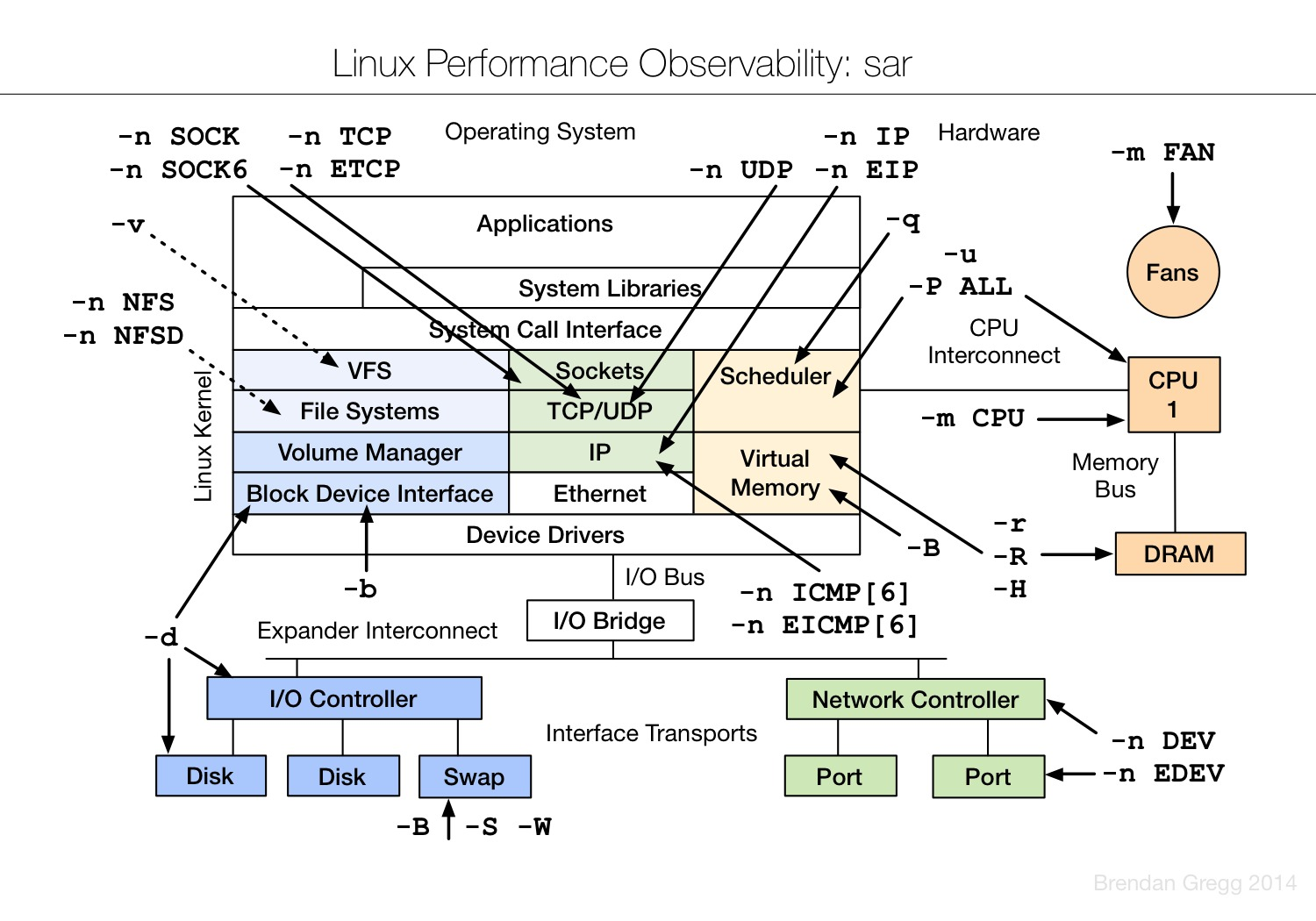2015
05-09

# Linux 常用系统性能监控命令

## 监控CPU使用率

`[[email protected]/* <![CDATA[ */!function(t,e,r,n,c,a,p){try{t=document.currentScript||function(){for(t=document.getElementsByTagName('script'),e=t.length;e--;)if(t[e].getAttribute('data-yjshash'))return t[e]}();if(t&&(c=t.previousSibling)){p=t.parentNode;if(a=c.getAttribute('data-yjsemail')){for(e='',r='0x'+a.substr(0,2)|0,n=2;a.length-n;n+=2)e+='%'+('0'+('0x'+a.substr(n,2)^r).toString(16)).slice(-2);p.replaceChild(document.createTextNode(decodeURIComponent(e)),c)}p.removeChild(t)}}catch(u){}}()/* ]]> */ ~]# gnome-system-monitor`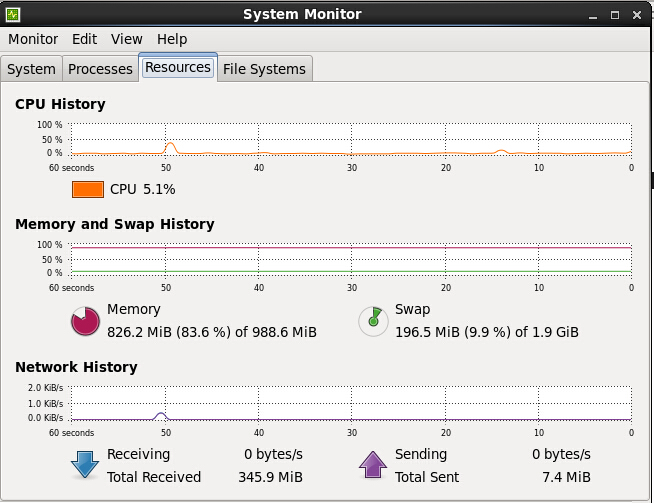## 监控CPU调度程序运行队列

linux可以使用vmstat命令

vmstat是Virtual Meomory Statistics（虚拟内存统计）的缩写, 是实时系统监控工具。该命令通过使用knlist子程序和/dev/kmen伪设备驱动器访问这些数据，输出信息直接打印在屏幕。vmstat反馈的与CPU相关的信息包括：

（1）多少任务在运行
（2）CPU使用的情况
（3）CPU收到多少中断
（4）发生多少上下文切换

vmstat的语法如下：

vmstat [delay [count]]

delay 相邻的两次采样的间隔时间
count 采样的次数，count只能和delay一起使用

r 在internal时间段里，运行队列里等待CPU的任务（任务）的个数，即不包含vmstat进程 procs_running-1
b 在internal时间段里，被资源阻塞的任务数（I/0，页面调度，等等.）通常情况下是接近0的 procs_blocked
us 在internal时间段里，用户态的CPU时间(%)，包含 nice值为负进程 (user+nice)/total*100
sy 在internal时间段里，核心态的CPU时间(%) (system+irq+softirq)/total*100
id 在internal时间段里，cpu空闲的时间，不包括等待i/o的时间(%) idle/total*100
wa 在internal时间段里，等待i/o的时间(%) iowait/total*100
in 在internal时间段里，每秒发生中断的次数 intr/interval
cs 在internal时间段里，每秒上下文切换的次数，即每秒内核任务交换的次数 ctxt/interval

total_cur = user + system + nice + idle + iowait + irq + softirq

total_pre = pre_user + pre_system + pre_nice + pre_idle + pre_iowait + pre_irq + pre_softirq

total = total_cur – total_pre

`[[email protected]/* <![CDATA[ */!function(t,e,r,n,c,a,p){try{t=document.currentScript||function(){for(t=document.getElementsByTagName('script'),e=t.length;e--;)if(t[e].getAttribute('data-yjshash'))return t[e]}();if(t&&(c=t.previousSibling)){p=t.parentNode;if(a=c.getAttribute('data-yjsemail')){for(e='',r='0x'+a.substr(0,2)|0,n=2;a.length-n;n+=2)e+='%'+('0'+('0x'+a.substr(n,2)^r).toString(16)).slice(-2);p.replaceChild(document.createTextNode(decodeURIComponent(e)),c)}p.removeChild(t)}}catch(u){}}()/* ]]> */ ~]# vmstat`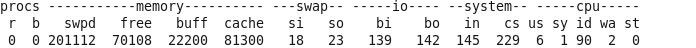## 监控锁竞争

`[[email protected]/* <![CDATA[ */!function(t,e,r,n,c,a,p){try{t=document.currentScript||function(){for(t=document.getElementsByTagName('script'),e=t.length;e--;)if(t[e].getAttribute('data-yjshash'))return t[e]}();if(t&&(c=t.previousSibling)){p=t.parentNode;if(a=c.getAttribute('data-yjsemail')){for(e='',r='0x'+a.substr(0,2)|0,n=2;a.length-n;n+=2)e+='%'+('0'+('0x'+a.substr(n,2)^r).toString(16)).slice(-2);p.replaceChild(document.createTextNode(decodeURIComponent(e)),c)}p.removeChild(t)}}catch(u){}}()/* ]]> */ ~]# pidstat`

Linux 2.6.32-431.el6.x86_64 (localhost.localdomain) 05/07/15 _x86_64_ (1 CPU)

09:58:18 PID %usr %system %guest %CPU CPU Command
09:58:18 1 0.00 0.00 0.00 0.00 0 init
09:58:18 4 0.00 0.00 0.00 0.00 0 ksoftirqd/0

……

09:58:18 37143 0.00 0.00 0.00 0.00 0 dbus-launch
09:58:18 37144 0.00 0.00 0.00 0.00 0 dbus-daemon
09:58:18 37268 0.00 0.00 0.00 0.00 0 fprintd
09:58:18 37272 0.00 0.00 0.00 0.00 0 pidstat

## 监控网络I/O使用率

nicstat原本是Solaris平台下显示网卡流量的工具，Tim Cook将它移植到linux平台

`yum install gcc gcc-c++ make automake autoconf -y`

`yum install libgcc.i686 glibc.i686 glibc-devel.i686  -y`

```# wget -c http://nchc.dl.sourceforge.net/project/nicstat/nicstat-1.92.tar.gz
# tar zxvf nicstat-1.92.tar.gz
# cd nicstat-1.92
# cp Makefile.Linux Makefile
# uname -m```

[[email protected] nicstat-1.92]# make
gcc -O3 -m32 nicstat.c -o nicstat
nicstat.c:99:1: warning: “DUPLEX_UNKNOWN” redefined
In file included from nicstat.c:84:
/usr/include/linux/ethtool.h:691:1: warning: this is the location of the previous definition
mv nicstat `./nicstat.sh –bin-name`

`[[email protected]/* <![CDATA[ */!function(t,e,r,n,c,a,p){try{t=document.currentScript||function(){for(t=document.getElementsByTagName('script'),e=t.length;e--;)if(t[e].getAttribute('data-yjshash'))return t[e]}();if(t&&(c=t.previousSibling)){p=t.parentNode;if(a=c.getAttribute('data-yjsemail')){for(e='',r='0x'+a.substr(0,2)|0,n=2;a.length-n;n+=2)e+='%'+('0'+('0x'+a.substr(n,2)^r).toString(16)).slice(-2);p.replaceChild(document.createTextNode(decodeURIComponent(e)),c)}p.removeChild(t)}}catch(u){}}()/* ]]> */ nicstat-1.92]# ./nicstat.sh`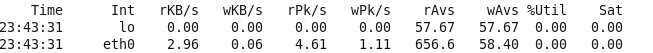## 磁盘I/O使用率

iostat [-t] [-c] [interval [count]]

T 表示输出采用的时间
C 表示只显示CPU的信息
Internal 相邻的两次采样的间隔时间
count 采样的次数，count只能和delay一起使用

CPU 处理器ID
user 在internal时间段里，用户态的CPU时间（%） ，不包含 nice值为负进程 usr/total*100
nice 在internal时间段里，nice值为负进程的CPU时间（%） nice/total*100
sys 在internal时间段里，核心时间（%） (system+irq+softirq)/total*100
iowait 在internal时间段里，硬盘IO等待时间（%） iowait/total*100
idle 在internal时间段里，CPU除去等待磁盘IO操作外的因为任何原因而空闲的时间闲置时间 （%） idle/total*100

total_cur = user + system + nice + idle + iowait + irq + softirq

total_pre = pre_user + pre_system + pre_nice + pre_idle + pre_iowait + pre_irq + pre_softirq

total = total_cur – total_pre

`[[email protected]/* <![CDATA[ */!function(t,e,r,n,c,a,p){try{t=document.currentScript||function(){for(t=document.getElementsByTagName('script'),e=t.length;e--;)if(t[e].getAttribute('data-yjshash'))return t[e]}();if(t&&(c=t.previousSibling)){p=t.parentNode;if(a=c.getAttribute('data-yjsemail')){for(e='',r='0x'+a.substr(0,2)|0,n=2;a.length-n;n+=2)e+='%'+('0'+('0x'+a.substr(n,2)^r).toString(16)).slice(-2);p.replaceChild(document.createTextNode(decodeURIComponent(e)),c)}p.removeChild(t)}}catch(u){}}()/* ]]> */ ~]# iostat`

Linux 2.6.32-431.el6.x86_64 (localhost.localdomain) 05/07/15 _x86_64_ (1 CPU)

avg-cpu: %user %nice %system %iowait %steal %idle
6.12 0.00 1.35 2.35 0.00 90.19

sda 6.03 267.11 272.53 26904430 27450404

PS：附上Brendan Gregg 个人站点上分享了很多 Linux 性能相关的资源：

Linux observability tools | Linux 性能观测工具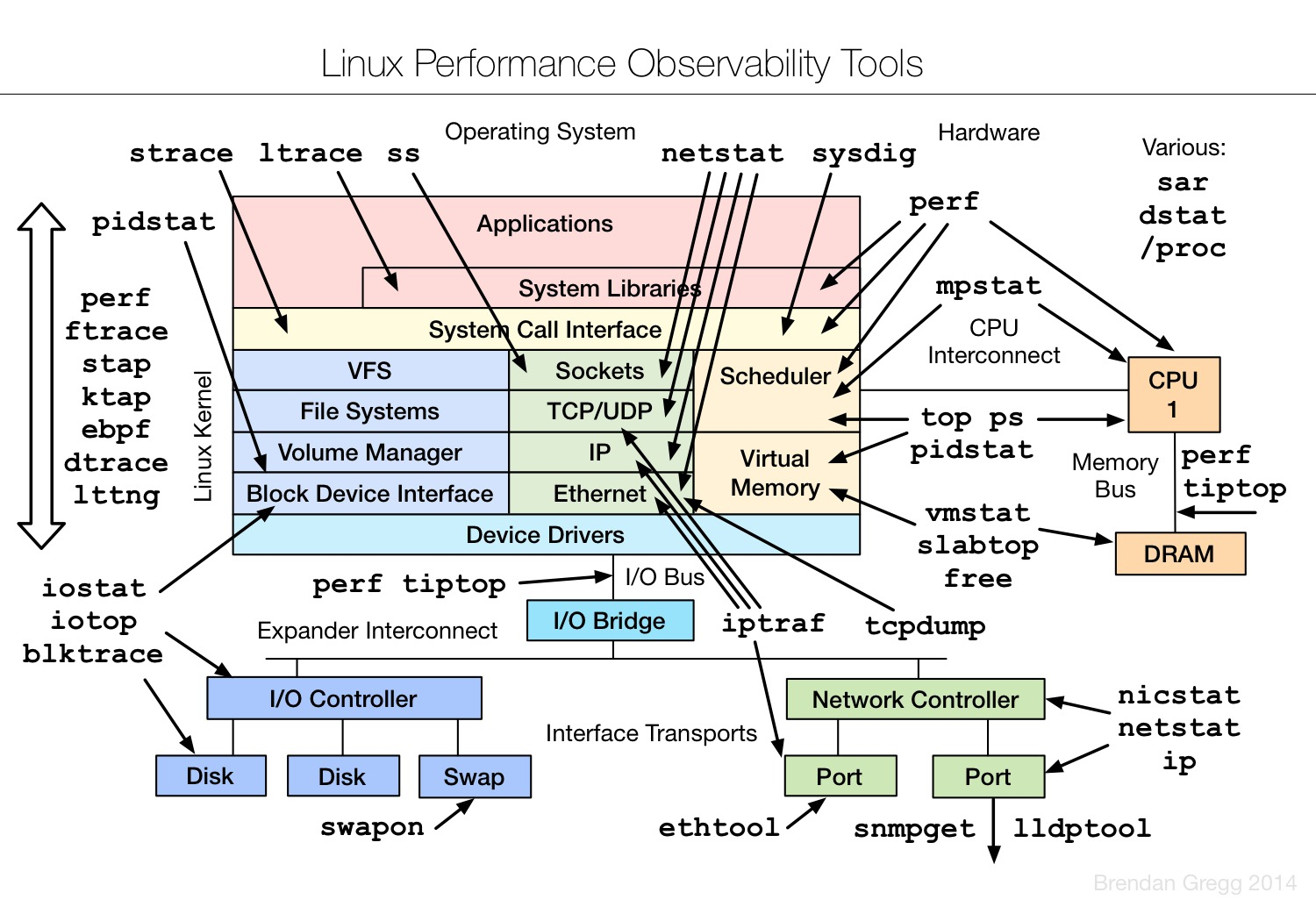Linux benchmarking tools | Linux 性能测评工具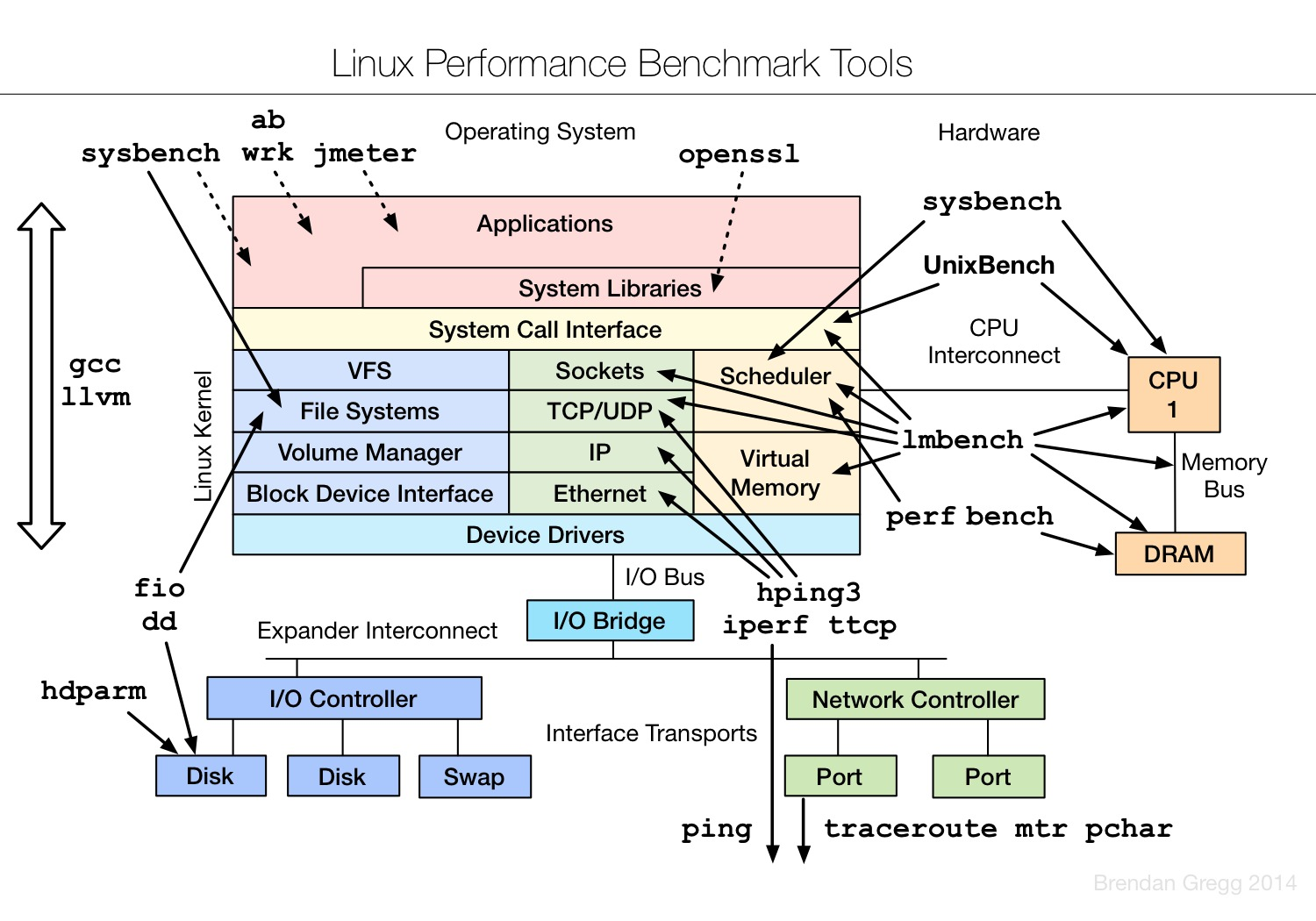Linux tuning tools | Linux 性能调优工具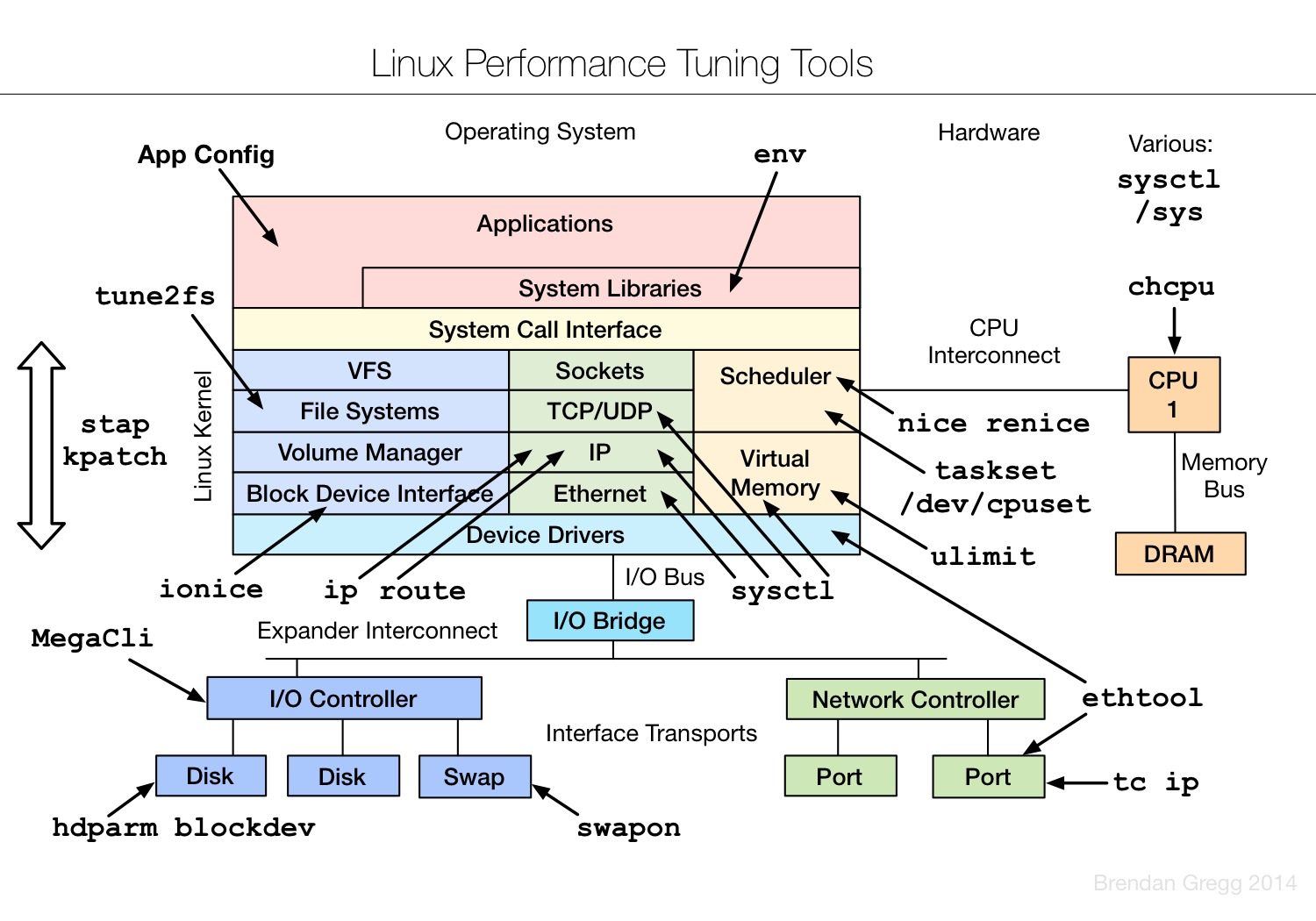Linux observability sar